RANDOM PAGE

SITE SEARCH

LOG
IN

HELP

# Op Amp Comparator

This is the AQA version closing after June 2019. Visit the the version for Eduqas instead.

## Comparator Behaviour

• Operational amplifiers are used in comparator subsystems.
• A comparator is a one bit Analogue to Digital Converter (ADC).
• A comparator compares two ANALOGUE input voltages.
• These are usually a reference voltage and a signal from a sensor.
• The output switches goes LOW or HIGH when the signal input crosses the reference voltage. This is a DIGITAL signal.
• The comparator relies on the very high open loop gain of the op' amp'. Very small changes in the input cause the op amp to saturate so the output is always low or high and almost never undecided.
• For a real-life op' amp' this gain will be between 105 and 107.
• This means that a potential difference between V1 and V2 of only a few microvolts is sufficient to saturate the op' amp'.

## Inverting and Non-Inverting Inputs

• Inverting: If the inverting input voltage rises above the other input, the op amp output voltage drops.
• Non-Inverting: If the non-inverting input voltage rises above the other input, the op amp output voltage also rises.
• With the very high open loop gain of the op amps, these output changes are very sudden and large.
• If V1 is greater than V2 then Vout will go high (close to the + supply voltage)
• If V1 is less than V2 then Vout will go low (close to the - supply voltage).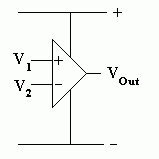## Simple Comparator Example - Practical Task

### Use this as a Practice Task for Writing Up Your Project

This is an example of the kind of work that should go into a top grade project. This has only one active device. You project will need at least three.

#### Specification

Turn on the LEDs when it gets dark.

• Run on +/- 10 to 15 Volts. Check this with a multimeter.
• Turn on the LEDs when the light level drops below 100 Lux. Check this with a Lux meter.
• Work correctly at 100 Lux at all voltages between 10 and 15 Volts. Use the Lux meter and multimeter to conduct this test.

#### How it Works

• MODULE 1
• R1 and R2 form a voltage divider.
• Since the resistors are equal, the voltage is divided equally and the potential at A is about 0V.
• MODULE 2
• R3 and the LDR form a potential divider.
• In the light, the LDR resistance is lower so the voltage at B rises.
• In the dark, the LDR resistance increases so the voltage at B drops.
• MODULE 3
• The 741 operational amplifier chip is being used as a comparator.
• The open loop gain of the op amp is very large (more than 100000).
• This means that tiny differences in the input voltages cause large changes in the op amp output.
• In bright light, the voltage at B is greater than the voltage sat A so the output of the op amp (C) is low (close to 0V). The op amp will be saturated.
• In dim light, the voltage at B is less than the voltage at A so the output of the op amp (C) is high (close to +12V). The op amp will be saturated.
• MODULE 4
• In the dark, the voltage at C is high (+12V) so about 10mA will flow through R4 and the LEDs will be lit.
• In bright light, the voltage at C is low (0V) so no current flows through the LEDs.
• Why Two LEDs?
• An ideal op amp would be able to produce output voltages of +12V and 0V.
• Real life op amps are not so good. They might only manage a high voltage of 9 to 12 V and a low voltage of 0 to 3 V.
• In this case, the low output voltage would still be enough to light a single LED even when it was not supposed to be lit. A fault like this could be fatal in an aircraft "Your Engine is On Fire" alert.
• By using two LEDs, each of which needs about 2V to light, four volts are needed to light the LEDs. This ensures that the LEDs go off when they are supposed to.

#### Circuit and Layout Diagram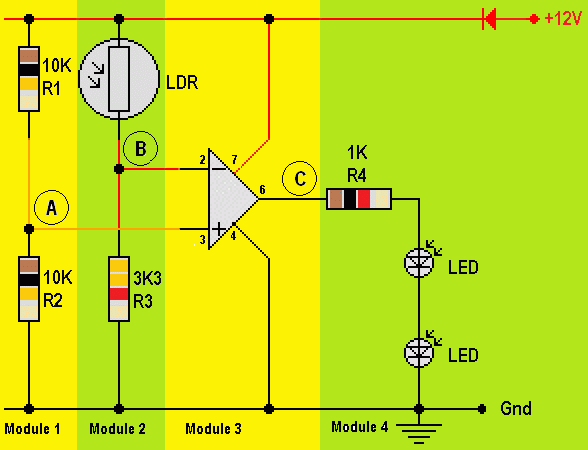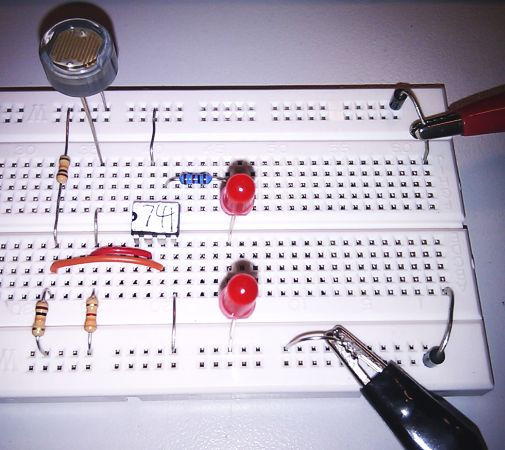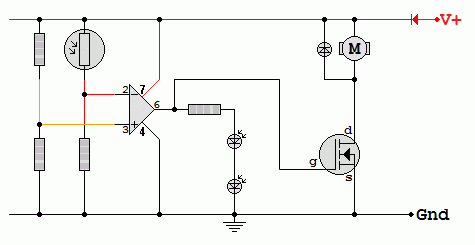## Simulation

For the Falstad Circuit Simulation, CTRL+Click Comparator
In options, check European Resistors and uncheck Conventional Current.
Use the slider to simulate changes in light level.

Alternatively view Op_Amp_Comparator.txt.
Save or copy the text on the web page. Import the saved or copied text into the Falstad simulator.

Here is the new HTML5 Simulator Site.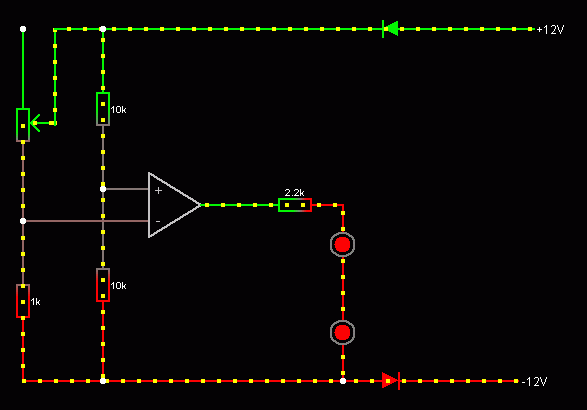reviseOmatic V3     Contacts, ©, Cookies, Data Protection and Disclaimers Hosted at linode.com, London